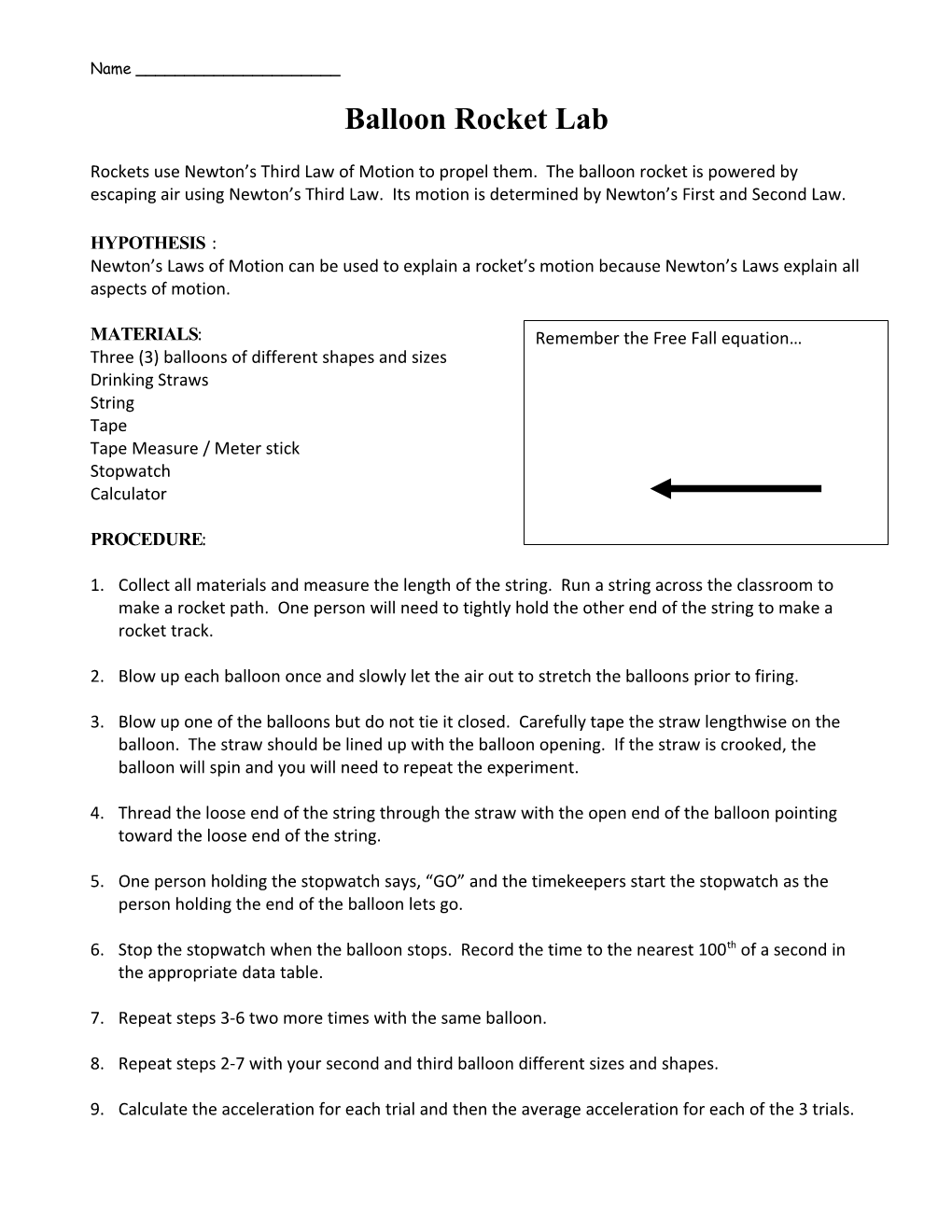# Balloon Rocket LabBalloon Rocket Lab

Rockets use Newton’s Third Law of Motion to propel them. The balloon rocket is powered by escaping air using Newton’s Third Law. Its motion is determined by Newton’s First and Second Law.

HYPOTHESIS:

Newton’s Laws of Motion can be used to explain a rocket’s motion because Newton’s Laws explain all aspects of motion.

MATERIALS:

Three (3) balloons of different shapes and sizes

Drinking Straws

String

Tape

Tape Measure / Meter stick

Stopwatch

Calculator

PROCEDURE:

1. Collect all materials and measure the length of the string. Run a string across the classroom to make a rocket path. One person will need to tightly hold the other end of the string to make a rocket track.
1. Blow up each balloon once and slowly let the air out to stretch the balloons prior to firing.
1. Blow up one of the balloons but do not tie it closed. Carefully tape the straw lengthwise on the balloon. The straw should be lined up with the balloon opening. If the straw is crooked, the balloon will spin and you will need to repeat the experiment.
1. Thread the loose end of the string through the straw with the open end of the balloon pointing toward the loose end of the string.
1. One person holding the stopwatch says, “GO” and the timekeepers start the stopwatch as the person holding the end of the balloon lets go.
1. Stop the stopwatch when the balloon stops. Record the time to the nearest 100th of a second in the appropriate data table.
1. Repeat steps 3-6 two more times with the same balloon.
1. Repeat steps 2-7 with your second and third balloon different sizes and shapes.
1. Calculate the acceleration for each trial and then the average acceleration for each of the 3 trials.

DATA:

First Balloon used: ______

1st Balloon / Trial 1 / Trial 2 / Trial 3 / Average
Time
Distance
Acceleration

Second Balloon used: ______

2nd Balloon / Trial 1 / Trial 2 / Trial 3 / Average
Time
Distance
Acceleration

Third Balloon used: ______

3rd Balloon / Trial 1 / Trial 2 / Trial 3 / Average
Time
Distance
Acceleration

QUESTIONS:

Answer the following questions. You must answer the questions using complete sentences. Thorough explanations are required.

1. Which balloon had the fastest acceleration? Which factors do you think contributed to the acceleration of the rocket?

______

1. Using the information you know and have reviewed about forces, draw a diagram showing all the forces acting on the balloon rockets.
1. Use Newton’s Three Laws of Motion to explain the motion of a balloon rocket from launch until it comes to a stop.

______

1. Can you think of three errors that happen in the experiment? How could you correct two out of those three?

______